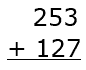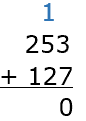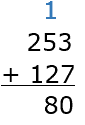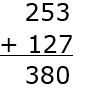## All About These 15 Worksheets

These Three Digit Addition worksheets are designed to help students master the concept of three-digit addition. The worksheets consist of column addition problems that require students to find either the sum or the missing addend of the equations involving three-digit numbers.

Each worksheet in the series includes a mix of problems with varying degrees of difficulty, designed to challenge and engage students at different skill levels. The problems are presented in a random order to prevent students from relying on memorization and encourage them to use their problem-solving skills.

These worksheets also provide ample space for students to show their work and write down their answers. This helps them develop their problem-solving skills and mental math abilities. This series is suitable for students in grades 2 to 4. It can be used in the classroom as a supplement to the math curriculum or at home as a way for parents to help their children practice and reinforce their math skills.

Overall, these Three Digit Addition worksheets provide a comprehensive and engaging way for students to master three-digit addition and develop their problem-solving skills. With these worksheets, students can build a solid foundation in math and develop the skills they need to succeed in higher-level math courses.

## How to Solve Three Digit Addition Problems

Performing three-digit addition problems involves adding numbers from right to left (ones place to hundreds place) while carrying over when necessary. Here’s a step-by-step guide that teachers can share with their students to help them in solving three-digit addition problems:

Step 1 – Write the Numbers

Write the numbers you want to add, aligning them vertically by their place values (ones, tens, and hundreds). Make sure the digits in each place value column line up.Step 2 – Add the Ones Place

Start by adding the digits in the ones place (the far right column). Write the sum below the line, in the same column. If the sum is greater than 9, write down the last digit and carry over the remaining digit(s) to the next column (tens place).Step 3 – Add the Tens Place

Add the digits in the tens place, along with any carry-over from the previous step. Write the sum below the line, in the same column. If the sum is greater than 9, write down the last digit and carry over the remaining digit(s) to the next column (hundreds place).Step 4 – Add the Hundreds Place

Add the digits in the hundreds place, along with any carry-over from the previous step. Write the sum below the line, in the same column.